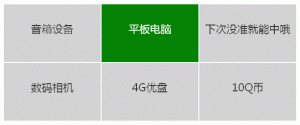PHP概率算法（适用于抽奖、随机广告）

HTML

<ul id="prize">
<li class="red" title="点击抽奖">1</li>
<li class="green" title="点击抽奖">2</li>
<li class="blue" title="点击抽奖">3</li>
<li class="purple" title="点击抽奖">4</li>
<li class="olive" title="点击抽奖">5</li>
<li class="brown" title="点击抽奖">6</li>
</ul>
<div><a href="#" id="viewother">【翻开其他】</a></div>
<div id="data"></div>

html结构中，我们使用一个无序列表放置6个不同的方块，每个li中的clas属性表示该方块的颜色，列表下面是一个链接a#viewother，用来完成抽奖后，点击它，翻看其他方块背面的中奖信息，默认是隐藏的。接下来还有一个div#data，它是空的，作用是用来临时存储未抽中的其他奖项数据，具体情况看后面的代码。为了让6个方块并排看起来舒服点，您还需要用CSS来美化下，具体可参照demo，本文中不再贴出css代码。

PHP

function get_rand(\$proArr) {
\$result = '';

//概率数组的总概率精度
\$proSum = array_sum(\$proArr);

//概率数组循环
foreach (\$proArr as \$key => \$proCur) {
\$randNum = mt_rand(1, \$proSum);
if (\$randNum <= \$proCur) {
\$result = \$key;
break;
} else {
\$proSum -= \$proCur;
}
}
unset (\$proArr);

return \$result;
}

\$prize_arr = array(
'0' => array('id'=>1,'prize'=>'平板电脑','v'=>1),
'1' => array('id'=>2,'prize'=>'数码相机','v'=>5),
'2' => array('id'=>3,'prize'=>'音箱设备','v'=>10),
'3' => array('id'=>4,'prize'=>'4G优盘','v'=>12),
'4' => array('id'=>5,'prize'=>'10Q币','v'=>22),
'5' => array('id'=>6,'prize'=>'下次没准就能中哦','v'=>50),
);

foreach (\$prize_arr as \$key => \$val) {
\$arr[\$val['id']] = \$val['v'];
}

\$rid = get_rand(\$arr); //根据概率获取奖项id

\$res['yes'] = \$prize_arr[\$rid-1]['prize']; //中奖项
unset(\$prize_arr[\$rid-1]); //将中奖项从数组中剔除，剩下未中奖项
shuffle(\$prize_arr); //打乱数组顺序
for(\$i=0;\$i<count(\$prize_arr);\$i++){
\$pr[] = \$prize_arr[\$i]['prize'];
}
\$res['no'] = \$pr;
echo json_encode(\$res);

jQuery

<script type="text/javascript" src="js/jquery-1.7.2.min.js"></script>
<script type="text/javascript" src="js/jquery-ui-1.7.2.custom.min.js"></script>
<script type="text/javascript" src="js/jquery.flip.min.js"></script>

\$(function(){
\$("#prize li").each(function(){
var p = \$(this);
var c = \$(this).attr('class');
p.css("background-color",c);
p.click(function(){
\$.getJSON("data.php",function(json){
var prize = json.yes; //抽中的奖项
p.flip({
direction:'rl', //翻动的方向rl：right to left
content:prize, //翻转后显示的内容即奖品
color:c,  //背景色
onEnd: function(){ //翻转结束
p.css({"font-size":"22px","line-height":"100px"});
p.attr("id","r"); //标记中奖方块的id
\$("#viewother").show(); //显示查看其他按钮
\$("#prize li").unbind('click')
.css("cursor","default").removeAttr("title");
}
});
\$("#data").data("nolist",json.no); //保存未中奖信息
});
});
});
});

\$(function(){
\$("#viewother").click(function(){
var mydata = \$("#data").data("nolist"); //获取数据
var mydata2 = eval(mydata);//通过eval()函数可以将JSON转换成数组

\$("#prize li").not(\$('#r')).each(function(index){
var pr = \$(this);
pr.flip({
direction:'bt',
color:'lightgrey',
content:mydata2[index], //奖品信息（未抽中）
onEnd:function(){
pr.css({"font-size":"22px","line-height":"100px","color":"#333"});
\$("#viewother").hide();
}
});
});
\$("#data").removeData("nolist");
});
});为什么我抽不到大奖？